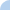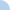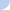AB Calculus: Differential Equations and growth equations

You may take this quiz as many times as you would like; I will only see the best result. There are 5 questions.

 This quiz is timed. The total time allowed for this quiz is 1 hour.Quiz Log In